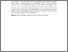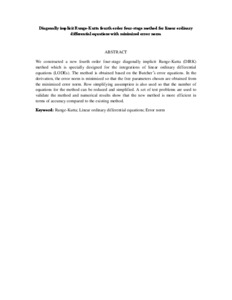# Diagonally implicit Runge-Kutta fourth order four-stage method for linear ordinary differential equations with minimized error norm

## Citation

Che Jawias, Nur Izzati and Ismail, Fudziah and Suleiman, Mohamed and Jaafar, Azmi (2009) Diagonally implicit Runge-Kutta fourth order four-stage method for linear ordinary differential equations with minimized error norm. Journal of Fundamental Sciences, 5 (1). pp. 69-78. ISSN 1823-626X

## Abstract

We constructed a new fourth order four-stage diagonally implicit Runge-Kutta (DIRK) method which is specially designed for the integrations of linear ordinary differential equations (LODEs). The method is obtained based on the Butcher’s error equations. In the derivation, the error norm is minimized so that the free parameters chosen are obtained from the minimized error norm. Row simplifying assumption is also used so that the number of equations for the method can be reduced and simplified. A set of test problems are used to validate the method and numerical results show that the new method is more efficient in terms of accuracy compared to the existing method.Preview
Text (Abstract)
Diagonally implicit Runge-Kutta fourth order four-stage method for linear ordinary differential equations with minimized error norm.pdfView Item